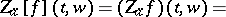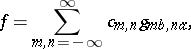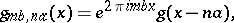# Zak transform

Jump to: navigation, search

Gel'fand mapping, $k$-$q$ representation, Weil–Brezin mapping

The Zak transform was discovered by several people in different fields and was called by different names, depending on the field in which it was discovered. It was called the "Gel'fand mapping" in the Russian literature because I.M. Gel'fand [a3] introduced it in his work on eigenfunction expansions associated with Schrödinger operators with periodic potentials. In 1967, almost 17 years after the publication of Gel'fand's work, the transform was rediscovered independently by a solid-state physicist, J. Zak, who called it the "k-q representation" . Zak introduced this representation to construct a quantum-mechanical representation for the motion of a Bloch electron in the presence of a magnetic or electric field [a8], [a9]. It has also been said [a7] that some properties of another version of the Zak transform, called the "Weil–Brezin mapping" in [a1], [a7], were even known to the mathematician C.F. Gauss. Nevertheless, there seems to be a general consent among experts in the field to call it the Zak transform, since Zak was indeed the first to systematically study that transform in a more general setting and recognize its usefulness.

The Zak transform $Z _ { a } ( f )$ of a function $f$ is defined by(a1)

\begin{equation*} = \sqrt { a } \sum _ { k = - \infty } ^ { \infty } f ( a t + a k ) e ^ { - 2 \pi i k w }, \end{equation*}

where $a > 0$ and $t$ and $w$ are real. When $a = 1$, one denotes $Z _ { a } f$ by $Z f$.

If $f$ represents a signal, then its Zak transform can be considered as a mixed time-frequency representation of $f$, and it can also be considered as a generalization of the discrete Fourier transform of $f$ in which an infinite sequence of samples in the form $f ( a t + a k )$, $k = 0 , \pm 1 , \pm 2 , \ldots$, is used (cf. also Fourier transform).

## Examples.

If $a = 1$ and $f ( t ) = 0$ outside $[ - b , b ]$, $0 < b \leq 1 / 2$, then $( Z f ) ( t , w ) = f ( t )$, $| t | \leq 1 / 2$.

The Zak transform of the Gaussian function

\begin{equation*} f ( t ) = ( 2 \gamma ) ^ { 1 / 4 } \operatorname { exp } ( - \pi \gamma t ^ { 2 } ) , \gamma > 0, \end{equation*}

is easily shown to be

\begin{equation*} ( Z f ) ( t , w ) = ( 2 \gamma ) ^ { 1 / 4 } e ^ { - \pi \gamma t ^ { 2 } } \theta _ { 3 } ( w - i \gamma t , e ^ { - \pi \gamma } ), \end{equation*}

where $\theta _ { 3 }$ is the third theta-function, defined by

\begin{equation*} \theta _ { 3 } ( z , q ) = \sum _ { k = - \infty } ^ { \infty } q ^ { k ^ { 2 } } e ^ { - 2 \pi i k z }. \end{equation*}

## Existence.

If $f$ is integrable or square integrable (cf. Integrable function), its Zak transform exists almost everywhere. In particular, if $f$ is a continuous function such that $| f ( t ) | \leq C ( 1 + | t | ) ^ { - (1 + \epsilon ) }$, for some $\epsilon > 0$, for all $t$, then its Zak transform exists and defines a continuous function.

## Elementary properties.

1) (linearity): for any complex numbers $a$ and $b$,

\begin{equation*} Z [ a f ( t ) + b g ( t ) ] ( t , w ) = a Z [ f ( t ) ] ( t , w ) + b Z [ g ( t ) ] ( t , w ). \end{equation*}

2) (translation): for any integer $m$,

\begin{equation*} Z [ f ( t + m ) ] ( t , w ) = e ^ { 2 \pi i m w } Z [ f ] ( t , w ); \end{equation*}

in particular,

\begin{equation*} ( Z f ) ( t + 1 , w ) = e ^ { 2 \pi i w } ( Z f ) ( t , w ). \end{equation*}

3) (modulation):

\begin{equation*} Z [ e ^ { 2 \pi i m t } f ] ( t , w ) = e ^ { 2 \pi i m t } ( Z f ) ( t , w ). \end{equation*}

4) (periodicity): The Zak transform is periodic in $w$ with period one, that is,

\begin{equation*} ( Z f ) ( t , w + 1 ) = ( Z f ) ( t , w ). \end{equation*}

5) (translation and modulation): By combining 2) and 3) one obtains

\begin{equation*} Z \left[ e ^ { 2 \pi i m t } f ( t + n ) \right] ( t , w ) = e ^ { 2 \pi i m t } e ^ { 2 \pi i n w } ( Z f ) ( t , w ). \end{equation*}

6) (conjugation):

\begin{equation*} ( Z \overline { f } ) ( t , w ) = \overline { ( Z f ) } ( t , - w ). \end{equation*}

7) (symmetry): If $f$ is even (cf. also Even function), then

\begin{equation*} ( Z f ) ( t , w ) = ( Z f ) ( - t , - w ), \end{equation*}

and if $f$ is odd, then

\begin{equation*} ( Z f ) ( t , w ) = - ( Z f ) ( - t , - w ). \end{equation*}

From 6) and 7) it follows that if $f$ is real-valued and even, then

\begin{equation*} ( Z f ) ( t , w ) = \overline { ( Z f ) } ( t , - w ) = ( Z f ) ( - t , - w ). \end{equation*}

Because of 2) and 4), the Zak transform is completely determined by its values on the unit square $Q = [ 0,1 ] \times [ 0,1 ]$.

8) (convolution): Let

\begin{equation*} h ( t ) = \int _ { - \infty } ^ { \infty } R ( t - s ) f ( s ) d s; \end{equation*}

then

\begin{equation*} ( Z h ) ( t , w ) = \int _ { 0 } ^ { 1 } ( Z R ) ( t - s , w ) ( Z f ) ( s , w ) d s. \end{equation*}

## Analytic properties.

If $f$ is a continuous function such that $f ( t ) = O \left( ( 1 + | t | ) ^ { - 1 - \epsilon } \right)$ as $| t | \rightarrow \infty$ for some $\epsilon > 0$, then $Z f$ is continuous on $Q$. A rather peculiar property of the Zak transform is that if $Z f$ is continuous, it must have a zero in $Q$. The Zak transform is a unitary transformation from $L ^ { 2 } ( \mathcal{R} )$ onto $L ^ { 2 } ( Q )$; see [a10], p. 481.

## Inversion formulas.

The following inversion formulas for the Zak transform follow easily from the definition, provided that the series defining the Zak transform converges uniformly (cf. also Uniform convergence):

\begin{equation*} f ( t ) = \int _ { 0 } ^ { 1 } ( Z f ) ( t , w ) d w , - \infty < t < \infty, \end{equation*}

\begin{equation*} \hat { f } ( - 2 \pi w ) = \frac { 1 } { \sqrt { 2 \pi } } \int _ { 0 } ^ { 1 } e ^ { - 2 \pi i w t } ( Z f ) ( t , w ) d t, \end{equation*}

and

\begin{equation*} f ( 2 \pi t ) = \frac { 1 } { \sqrt { 2 \pi } } \int _ { 0 } ^ { 1 } e ^ { - 2 \pi i x t } ( Z \widehat { f } ) ( x , t ) d x, \end{equation*}

where $\hat { f }$ is the Fourier transform of $f$, given by

\begin{equation*} \hat { f } ( w ) = \frac { 1 } { \sqrt { 2 \pi } } \int _ { - \infty } ^ { \infty } f ( x ) e ^ { i w x } d x. \end{equation*}

## Applications.

The Zak transform has been used successfully in various applications in physics, such as in the study of the coherent states representation in quantum field theory [a6], and in electrical engineering, such as in time-frequency representation of signals and in digital data transmission; see [a4], [a5].

The applications of the Zak transform are not limited to only physics and engineering. More recent applications of it in mathematics have proved to be very useful; in particular, to simplify proofs of some important results. A case in point is the Gabor representation problem. The Gabor representation problem can be stated as follows: Given $g \in L ^ { 2 } ( \mathcal{R} )$ and two real numbers, $a$, $b,$ different from zero, is it possible to represent any function $f \in L ^ { 2 } ( \mathcal{R} )$ by a series of the formwhere $g_{mb , na}$ are the Gabor functions, defined byand $c_{m,n}$ are constants? And under what conditions is the representation unique?

The Zak transform has been used successfully to study the orthogonality and the completeness of Gabor frames in the crucial case where $a b = 1$; see [a2], [a10].

How to Cite This Entry:
Zak transform. Encyclopedia of Mathematics. URL: http://encyclopediaofmath.org/index.php?title=Zak_transform&oldid=50696
This article was adapted from an original article by Ahmed I. Zayed (originator), which appeared in Encyclopedia of Mathematics - ISBN 1402006098. See original article